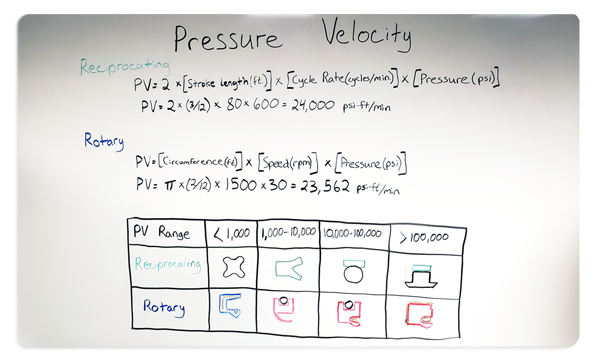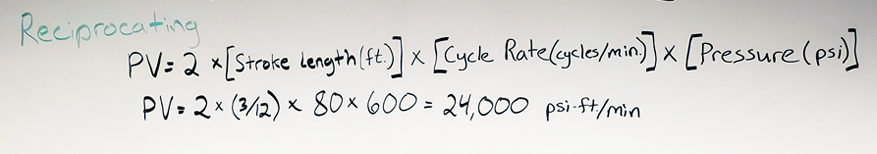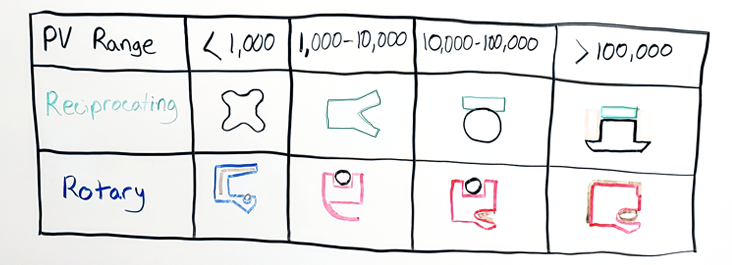1.866.412.4880# What is Pressure Velocity## Whitepapers On Whiteboard

Expert Level
Intermediate
Length
4:25
Instructor
Jason Huff## Summary

Pressure velocity, or PV value, is the combination of the pressure of the application and the speed of either the rotating or reciprocating shaft. The PV limit is the maximum value of that combination where the seal will function and wear normally. If we exceed that value, we’re going to see excessive wear which will lead to sealing failure.

There are several factors to consider when selecting a seal. Each factor has a direct impact on the performance and lifespan of your application. One of the most significant, but often overlooked, is the pressure-velocity, or PV, of your seal.

Jason Huff spends some time defining pressure-velocity, the calculations, and walking through examples to show its significance.Click on the image to open in a New Tab.

## Video Transcript

When selecting a seal, there are several factors that we need to consider. Including pressure, speed, temperature, the media you’re trying to seal, the hardness, and surface finish of the mating hardware.

And arguably one of the most important things that we need to take into consideration is the PV value or pressure velocity.

This is the combination of the pressure of the application and the speed of either the rotating or reciprocating shaft. The PV limit is the maximum value of that combination where the seal will function and wear normally. If we exceed that value, we’re going to see excessive wear which will lead to sealing failure.

## Reciprocating PV CalculationFor a reciprocating application, to calculate the PV value:

• take the stroke length in feet
• multiply that by the cycle rate in cycles per minute
• multiply that by the pressure in PSI

## Reciprocating ExampleIf we had an application that had a stroke length of 3-inches and a cycle rate of 80 cycles per minute and a pressure of 600 PSI:

• 600 PSI should be no problem for a quad ring
• A u-cup will handle 600 PSI – no problem
• And then obviously these two versions of a cap seal can handle 600 PSI

The issue becomes when we combine that with the speed of 80 cycles per minute, which is fast for a reciprocating application.

We’re going to take our:

• three-inch stroke length divide that by 12 to get it in feet
• multiply that by 2 to capture the entire distance traveled
• multiply the 80 cycles per minute
• multiply 600 PSI

That puts our PV value at 24,000.

When we reference our seal selection chart you can see both the quad ring and u-cup are no longer viable options and we’re going to have to stick to one of these cap seal options.

## Rotary PV CalculationSimilarly, if we want to calculate the PV value for a rotary application, we’re going to take:

• the circumference of our shaft in feet
• multiply that by the speed in RPM
• multiply that by the pressure in PSI

## Rotary ExampleIf we had a 2-inch diameter shaft, and it was rotating at 1500 RPM and a pressure of 30 PSI:

• 1500 RPM for a traditional rotary lip seal – no problem
• A Flexi-lip or PTFE lip seal – no problem
• The same with these spring energized PTFE seals

Now that we have to consider 30 PSI that automatically puts are rotary lip seal out because that’s exceeding its max range – 30 PSI for the PTFE lip seal is no problem. Not a problem for the spring energized PTFE seals either.

But, when we combine the two:

• our 2-inch shaft divided by 12 so that we’re in units of feet
• multiply that by pi to get the circumference
• multiply 1500 RPM
• multiply 30 PSI

That puts our PV value at 23,562.

Again, now it eliminates those first two options as being acceptable seals.

## Summary

It’s very important to not only consider the pressure and velocity independently – we need to combine the two so that we get a true understanding of what the seal is going to see in application.